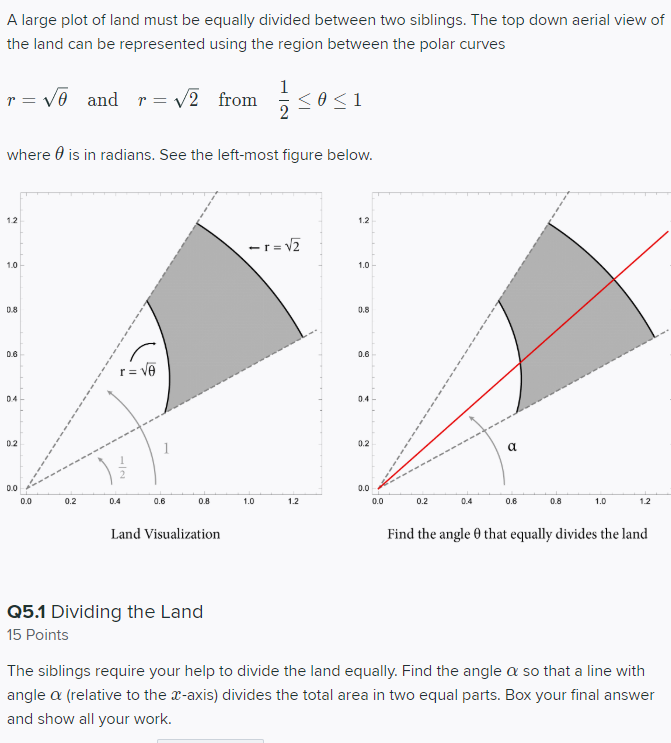# Question A large plot of land must be equally divided between two siblings. The top down aerial view of the land can be represented using the region between the polar curves prvo and r=v2 from sosi where is in radians. See the left-most figure below. -I = V2 0.0 0.0 0.2 0.4 0.6 0.8 1.0 1.2 0.0 0.2 0.4 0.6 0.8 1.0 1.2 Land Visualization Find the angle that equally divides the land Q5.1 Dividing the Land 15 Points The siblings require your help to divide the land equally. Find the angle a so that a line with angle a (relative to the c-axis) divides the total area in two equal parts. Box your final answer and show all your work.